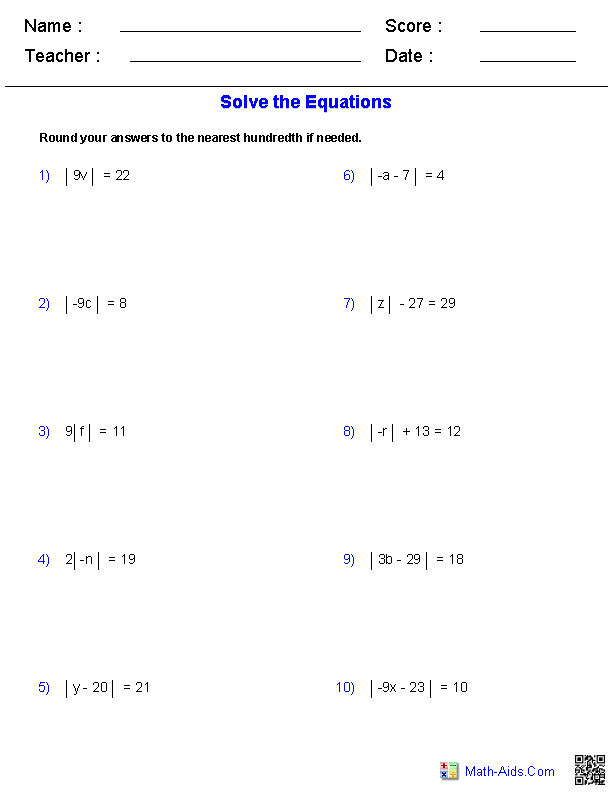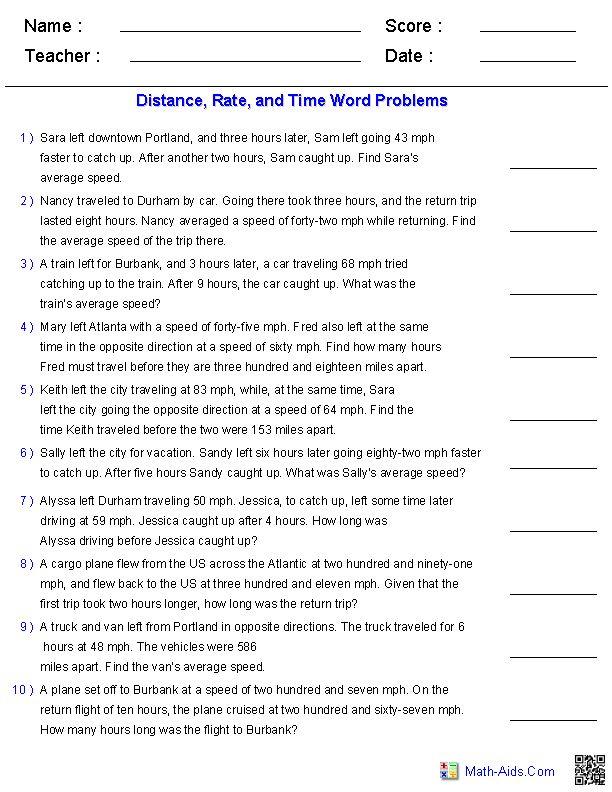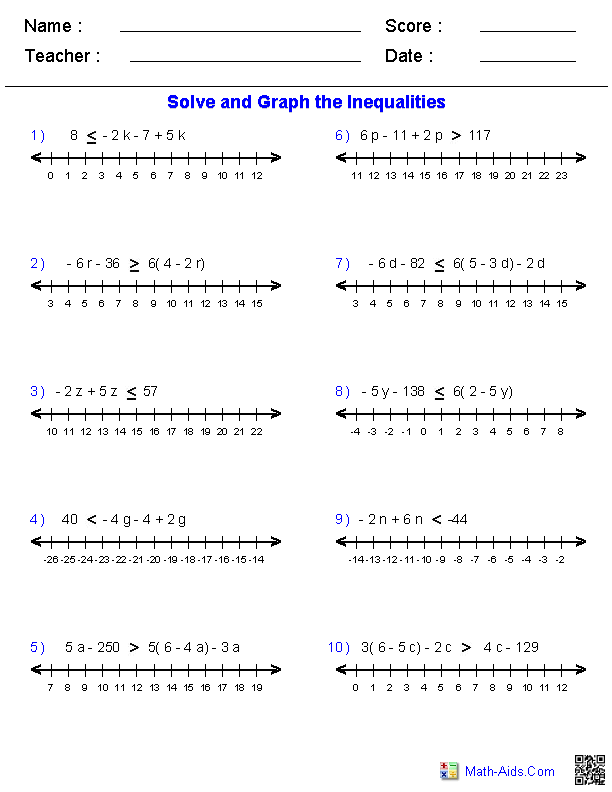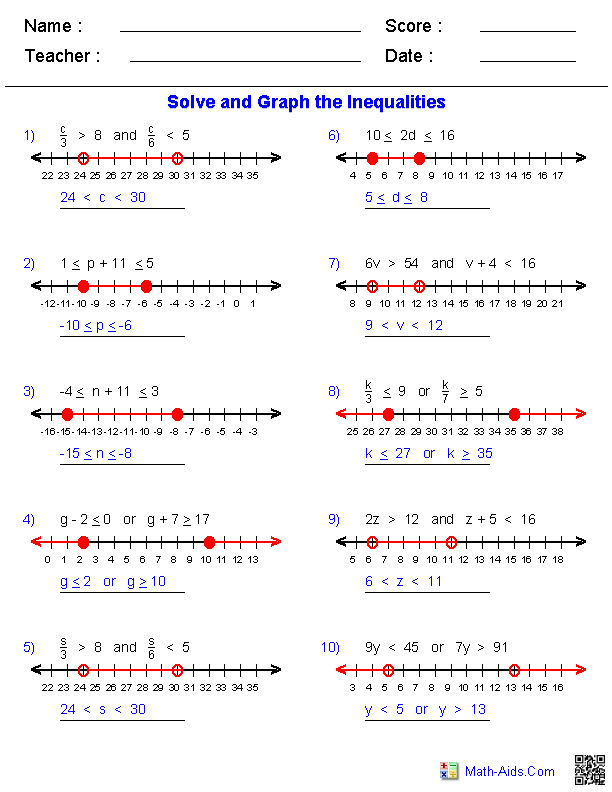#Algebra 2 Worksheets

## Equations and Inequalities Worksheets

Here is a graphic preview for all of the Equations and Inequalities Worksheets. You can select different variables to customize these Equations and Inequalities Worksheets for your needs. These Equations and Inequalities Worksheets are randomly created and will never repeat so you have an endless supply of quality Equations and Inequalities Worksheets to use in the classroom or at home.

Our Equations and Inequalities Worksheets are free to download, easy to use, and very flexible.

These Equations and Inequalities Worksheets are a good resource for students in the 8th Grade through the 12th Grade.

Click here for a Detailed Description of all the Equations and Inequalities Worksheets.

## Quick Link for All Equations and Inequalities Worksheets

Click the image to be taken to that Equations and Inequalities Worksheets.

##### Multiple Step EquationsIntegers Worksheets##### Multiple Step EquationsDecimals Worksheets##### Absolute ValueEquations Worksheets##### Distance, Rate, and TimeWord Problems##### Mixture Word ProblemsEquations Worksheets##### Work Word ProblemsEquations Worksheets##### Multiple Step InequalitiesInequalities Worksheets##### Compound InequalitiesInequalities Worksheets##### Absolute Value InequalitiesInequalities WorksheetsRecommended Videos

## Detailed Description for All Equations and Inequalities Worksheets

Multiple Step Equations with Integers Worksheets
These Equations and Inequalities Worksheets will produce multiple step problems containing integers. These worksheets will produce ten problems per worksheet. These Equations and Inequalities Worksheets are a good resource for students in the 8th Grade through the 12th Grade.

Multiple Step Equations with Decimals Worksheets
These Equations and Inequalities Worksheets will produce multiple step problems containing decimals. These worksheets will produce ten problems per worksheet. These Equations and Inequalities Worksheets are a good resource for students in the 8th Grade through the 12th Grade.

Absolute Value Equations Worksheets
These Equations and Inequalities Worksheets will produce absolute value problems with monomials and polynomials expressions. These worksheets will produce ten problems per worksheet. These Equations and Inequalities Worksheets are a good resource for students in the 8th Grade through the 12th Grade.

Distance, Rate, and Time Word Problems
These Equations and Inequalities Worksheets will produce distance, rate, and time word problems with ten problems per worksheet. You may select the numbers to be represented with digits or in words. These Equations and Inequalities Worksheets are a good resource for students in the 8th Grade through the 12th Grade.

Mixture Word Problems
These Equations and Inequalities Worksheets will produce mixtures word problems with ten problems per worksheet. You may select the numbers to be represented with digits or in words. These Equations and Inequalities Worksheets are a good resource for students in the 8th Grade through the 12th Grade.

Work Word Problems
These Equations and Inequalities Worksheets will produce work word problems with ten problems per worksheet. You may select the numbers to be represented with digits or in words. These Equations and Inequalities Worksheets are a good resource for students in the 8th Grade through the 12th Grade.

Multiple Step Inequalities Worksheets
These Equations and Inequalities Worksheets will produce multiple step inequalities problems for graphing. You may select which type of inequality to use in the problems. These Equations and Inequalities Worksheets are a good resource for students in the 8th Grade through the 12th Grade.

Compound Inequalities Worksheets
These Equations and Inequalities Worksheets will produce compound inequalities problems for graphing. You may select which type of inequality to use in the problems. These Equations and Inequalities Worksheets are a good resource for students in the 8th Grade through the 12th Grade.

Absolute Value Inequalities Worksheets
These Equations and Inequalities Worksheets will produce absolute value inequalities problems for graphing. You may select which type of inequality to use in the problems. These Equations and Inequalities Worksheets are a good resource for students in the 8th Grade through the 12th Grade.